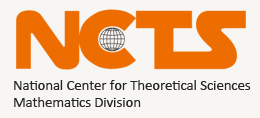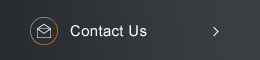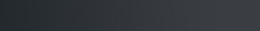Sponsored by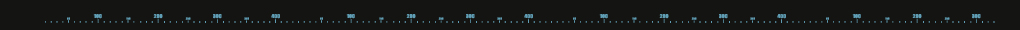[ Events ]

﻿
Activity Search
Sort out
 select all Seminars and Talks Conference and Workshop Lectures and Courses Else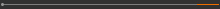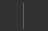Field
 select all Number Theory and Representation Theory Algebraic Geometry Differential Geometry and Geometrical Analysis Differential Equations and Stochastic Analysis Scientific Computing Interdisciplinary Studies Laboratory of Data Science Harmonic Analysis OthersYear
 select 2015 2016 2017 2018 2019 2020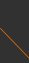NCTS Seminar on Scientific Computation14:30 - 15:20, August 7, 2019 (Wednesday) SA223, Science Building I, NCTU (交通大學科學一館 223室) Newton’s Method for Coupled Continuous-Time Algebraic Riccati Equations Eric King-Wah Chu (Monash University) Abstract: We are interested in the solution of the coupled continuous-time algebraic Riccati equation, arising in the optimal control of Markovian jump linear systems. Newton's method is applied to construct the solution, leading to some coupled Lyapunov equations. Several iterative methods of \$O(n^3)\$ computational complexity for the coupled Lyapunov equation and Newton's method for the coupled continuous-time algebraic Riccati equation have been analyzed . Illustrative examples will be presented.(C) 2019 National Center for Theoretical Sciences